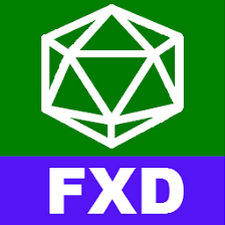FX Draw Tools is available as a free download from our software library. FX Draw provides a high-productivity drawing environment designed specifically for mathematics teachers. Quickly create editable, high-quality diagrams for tests, exams, worksheets, web sites, presentations and demonstrations.

FX Sketch, the freehand extension of FX Draw, lets you sketch mathematical diagrams and have them automatically converted to professional quality drawings that can be edited using the power of FX Draw.

Efofex also provides FX Draw subscribers with three, free bonus programs that provide simplified access to some of FX Draw’s powerful graphing and equation tools. You can use the bonus tools with students or to rapidly create equations for use in documents.Geometric Diagrams:

• Inbuilt geometry system that understands tangents, perpendiculars, parallel lines, common tangents, angle bisectors and equal lengths lines. Makes drawing geometric diagrams intuitive.
• Inbuilt geometry system that understands tangents, perpendiculars, parallel lines, common tangents, angle bisectors and equal lengths lines. Makes drawing geometric diagrams intuitive.
• Multiple tools to draw lines including end points, mid points, rise/run and length/angle.
• Over twenty arrow types for lines including parallel line marks and circles.
• Multiple tools to draw circles including centre/point on circumference, diameter, circle defined by three points and circle inscribed in square.
• Compass like drawing tools. Can be used to simulate geometric constructions.
• Quickly mark and annotate angles with a selection of ten different mark types.
• Six specialized triangle drawing tools that allow you to quickly draw any triangle.
• Triangles understand in-centres, circum-centres, centroids and orthocentres.
• Seven specialized quadrilateral drawing tools that allow you to quickly draw rectangles, kites, parallelograms, rhombuses and trapeziums.
• Highly efficient point-marking tool to mark and annotate important points on your geometric diagram.
• Option to automatically mark and annotate important points when drawing geometric diagrams.
• Multiple arc and ellipse tools that allow you to construct exactly the arc/ellipse you need.
• Regular polygon tools that allow you to draw squares to regular 30000-gons.

Drawing Tools:

• 180 and 360 degree protractors that can be added to any diagram
• A ruler tool that allows you to show accurate measurements. The ruler can be totally customised including negative values.
• Bitmaps, PNG and JPEG files can be pasted into FX Draw. This allows you to use FX Draw’s specialized mathematical tools to annotate real situations.
• Draw Bezier curves and smoothed lines.
• Advanced dynamic flood fill tool.
• Free rotation and reflection of objects.

Text/Equation Entry:

• FX Draw includes our FX Autoquate technology which allows you to easily mix equations and normal text. The system automatically detects which bits need to be formatted as equations.
• FX Draw includes our full FX Equation product which makes equation entry as quick as typing.

Function Graphs:

• FX Draw includes our full FX Graph graphing tool which allows you to quickly create and annotate any graph.
Quick, visual function entry system that allows you to simply type the function but shows you exactly how it is interpreting your entry.
• Graph Cartesian functions, inverse functions, polar functions, parametric functions, implicitly defined functions, points, vectors, Argand diagrams, slope fields, piecewise defined functions, domains, inequations and feasible regions.
• Show families of curves, demonstrate transformational graphing and create animations.

Statistical Graphs:

• FX Draw includes our full FX Stat graphing tool which allows you to quickly create statistical data and graphs.
• Create data sets which fit fifteen different probability functions, including time-series data and bivariate data.
• The data generators allow you to quickly create questions.
• Automatically calculate summary statistics for data sets.
• Graph raw data, grouped data and frequency tables.
• Draw column graphs, bar graphs, pie graphs, box and whisker plots, cumulative frequency graphs, dot plots, stem and leaf diagrams, line graphs, frequency polygons, histograms, cumulative histograms, back-to-back histograms, divided bar graphs, scatterplots, residuals graphs, link graphs and triangle graphs.

Specialized Tools:

• Bearings diagram tool.
• Normal distribution drawing tool.
• Number line tool. Vertical and horizontal numbers. Draw x = a, x > a, x < a, x = a and x a.
• Two and three circle Venn diagrams with easy shading of regions.
• Graph paper tool. Create Cartesian, isometric, hexagonal and polar grids and dot papers. Create log and log/log papers.
• Unit circle tool.
• Tree diagram drawing tool.
• Clock tool. Draw fully customisable clock faces with or without hands.
• Isometric drawings. Create an isometric grid that allows you to easily create isometric drawings.

Gallery of Objects:

• FX Draw is supplied with hundreds of predrawn, gallery objects
• Galleries include chance processes, circle geometry, dominoes, environmental images, hexominoes, isometric diagrams, Menger’s sponge, optical illusions, pentominoes, Platonic solids, prisms, pyramids, cones, cylinders, pulley systems, regular polygons, Sierpinksi’s triangle, the snowflake curve, simultaneous equations, spheres, hemispheres, latitude/longitude problems, tangrams, tesselations, triangles and trigonometry diagrams
• Each gallery image is dynamic and fits the available space. More flexible than clipart
• Gallery images can be broken into component parts

Output Options:

• High resolution bitmaps, PNG, TIF and JPG files
• Vector based SVG files
• Insertable OLE object

Integration and Compatibility:

• One file type compatible across all platforms
• Connection based system which allows integration with any product
• Insertable OLE object
• Integration with Word 2007 (and later) ribbon bar
• Integration with Powerpoint 2010 (and later) ribbon bar
• Output suitable for Pages and Keynote
• Create FX Draw file from multiple inserted OLE objects in a Word file
Title: FX Draw Tools v19.10.2019
Developer: Efofex Software
Language: English
OS: Windows 7/8/8.1/10.
– Fix issue with rendering of Stem&Leaf diagrams in countries which use commas as decimal points.
– Improve rendering of large brackets and integral signs